In a previous post, we met the Mandelbrot set by plotting it crudely in the console, as illustrated below.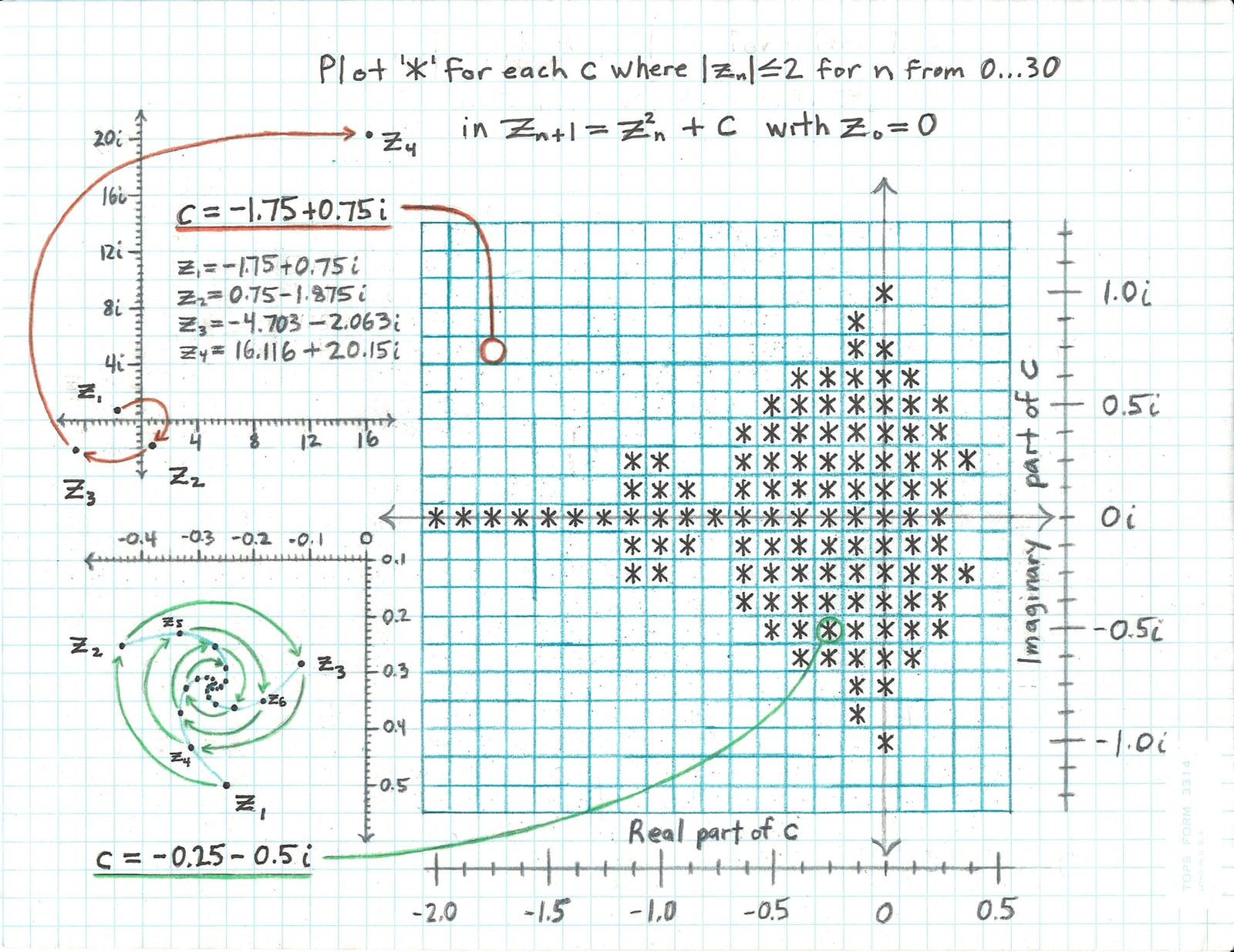We mapped a grid of characters over the complex plane, printing * in grid cells that mapped to complex points belonging to the Mandelbrot set, and space characters in cells that didn’t. The grid in the illustration is a mere 21 × 21 characters, because I didn’t want to have to draw any more than that!

Recall that a point in the complex plane belongs to the Mandelbrot set if, when it is substituted for $$c$$ in

$z_{n+1} = z_n^2+c,$

with $$z_0 = 0$$, none of the $$z_n$$ has a magnitude—a distance from the origin at $$0 + 0i$$—greater than $$2$$. In other words, when $$\lvert z_n \rvert$$ is bounded by $$2$$, $$c$$ is in the set; otherwise, it’s not. For the plot above, no more than 30 iterations were performed to determine boundedness for each $$c$$.

The illustration shows two examples of $$c$$. For $$c = -1.75 + 0.75i$$, $$\lvert z_n \rvert$$ rapidly exceeds $$2$$. But for $$c = -0.25 - 0.5i$$, $$\lvert z_n \rvert$$ is always less than $$2$$, and $$z_n$$ approaches something like $$-0.28 - 0.32i$$, with the sequence of values plotting out a surprisingly elegant three-armed spiral galaxy along the way.

To see the set in higher resolution, we need to shrink those grid cells down from the size of a character in the console to the size of a pixel in an image. Then, instead of choosing different characters to indicate whether the corresponding point in the complex plane belongs to the set, we’ll choose different colors.

A few thoughts come to mind.

First: a nice big image on a modern computer will have a lot of pixels. The most points we plotted in the console was 220 × 55, or 12,100. To determine the boundedness of the iterated Mandelbrot function for each point, we have to compute several floating point operations in each of potentially dozens of iterations. Let’s make a rough estimate that our largest console plots required a hundred floating point ops for each of ten thousand points, for total of a million floating point ops. That’s not hard for a modern CPU. We can time the final console plot like so:

let start = CFAbsoluteTimeGetCurrent()
let bestMandelPoints = ComplexPlotPoints(from: Complex(-3.0, 1.0), to: Complex(1.0, -1.0), horizontalPointCount: 220, verticalPointCount: 55)

let bestMandelplot = bestMandelPoints.map { points in
points.map { point in
plotValue(for: IteratedFuncValues(f: f(c: point), iterations: 30).bounded)
}
}

print("Best mandelplot!")
for row in bestMandelplot {
print(String(row))
}
let end = CFAbsoluteTimeGetCurrent()
print(end - start)


Including outputting to the console, I find it takes an average of 0.13 seconds on my 2018 15” MacBook Pro. Its Intel Core i7-8750H has a maximum single-core frequency of 4.1 GHz. And that code is not optimized.

But making high-resolution images of the Mandelbrot set is something else. My MacBook’s native screen resolution is 2880 × 1800 pixels—a total of more than 5 million! And (spoiler alert) we’ll find that in some cases we want to compute not dozens, but hundreds of iterations of the Mandelbrot function per point. Even for an image that doesn’t fill the whole screen, that’s more like a thousand floating point ops for each of a few million pixels: a few billion total floating point ops!

All other things being equal (they aren’t, but we’re just doing a Fermi estimate), a high-resolution image of the Mandelbrot set will take a few thousand times longer to render than our console plots. On my computer, the console plots took about a tenth of a second, so we’re talking about a few hundred seconds per image.

That doesn’t bode well for the second thought that comes to mind: I’d really like to do more than just generate static images of the Mandelbrot set. I’d like to interactively explore it. I want an app that will let me pan around and zoom into the set, and modern apps set the expectation that we can do that at 60 frames per second. Certainly not hundreds of seconds per frame. But now we’re talking about hundreds of billions of floating point operations per second!

And there’s another problem with making an interactive Mandelbrot set exploration app—a personal one for me, anyway: whether we’re talking about a macOS or iOS app, I don’t know what API allows setting the value of an individual pixel in a view.

However, there may be a solution to both the performance problem and my ignorance of how to plot a pixel directly. Rendering the Mandelbrot set is an embarrassingly parallel1 task. The set membership of each point in the complex plane can be computed independently of every other point, as the only data needed for the computation are the real and imaginary components of $$c$$. If we wanted to render a 10000 × 10000 pixel—a 100 megapixel!—image of the set, and we had a hundred-million-core CPU, we could compute the values of each of those pixels simultaneously.

Hundred-million-core CPUs are thin on the ground here in 2020, but pretty much every computer these days has a GPU, and they have lots of cores2 that can do floating point computations. My MacBook’s AMD Radeon Pro 555X has 768 of them.3 Let’s investigate the feasibility of exploring the Mandelbrot set with a GPU!

We just estimated that to render pans and zooms of a few-megapixel image of the Mandelbrot set at 60 frames per second, we’d need the ability to complete a few hundred billion floating point operations per second, or roughly 200 GFLOPS. The Radeon Pro 555X has a theoretical performance with 32-bit floating point values of nearly 1400 GFLOPS.3 That gives us some headroom.

However, we used a 64-bit floating point type, Swift’s Double, to plot the Mandelbrot set in the console. And when we explored the emergence of Pi in the set, we learned that as we zoom further into it, we need ever more bits in our floating-point values to avoid rounding errors. The 555X’s 64-bit floating point performance is much lower, at about 87 GFLOPS. That won’t give us 60 fps animations of the set. Will 32-bit computation still provide enough accuracy, in exchange for much greater performance? Let’s find out.

First, how will this work, exactly? GPUs were originally designed to accelerate the rendering of 3D graphics. How will that help us render 2D images of the Mandelbrot set? To find out, let’s review the basics of 3D rendering.4

3D scenes are built up from 3D models, which are in turn are built from many 2D geometric primitives—mostly triangles. As an extremely simple example, a pyramid could be modeled out of four triangles—one for each side—and a square for its base. And since most 3D hardware doesn’t support rectangles directly, by the time it gets to the GPU, the square will probably have been decomposed into two right triangles joined at their hypotenuses.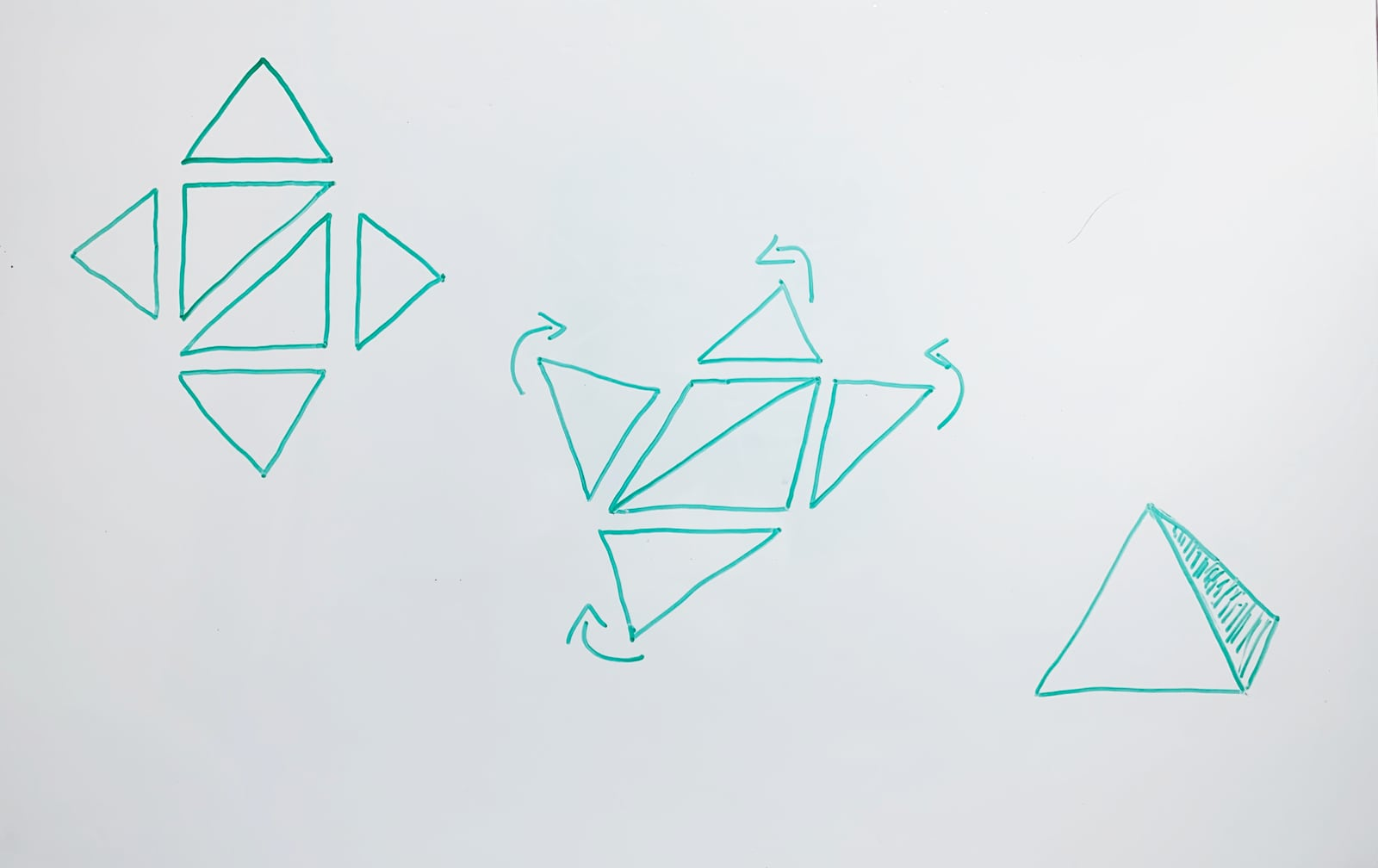The geometric primitives making up a model can be concisely defined by their vertices: the points in 3D space where their edges come together. A triangle has three vertices at the points where its three edges join. And being a point in 3D space, a vertex can be defined by three numbers: its $$x$$, $$y$$, and $$z$$ coordinates. The following diagram shows the coordinates of each vertex of a triangle living in a 3D coordinate space I made up.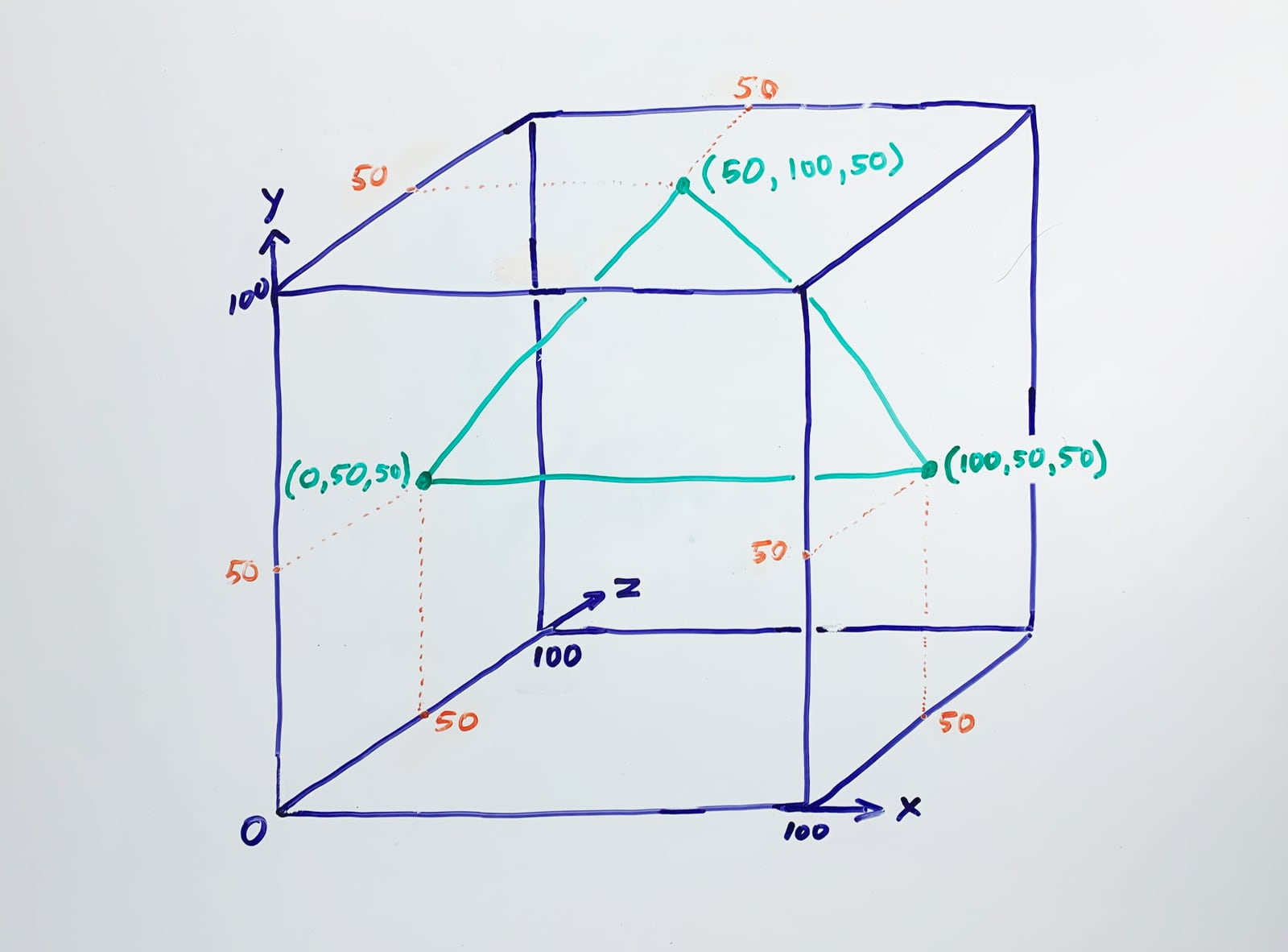Objects in an animated 3D scene are made to move and change shape by recalculating the coordinates of the vertices of their polygons. As highly-detailed scenes comprise millions or even billions of vertices, animating them requires a lot of computation!

But manipulating lots of vertices inside the GPU isn’t very satisfying for human computer users. We want to see it! That is accomplished in the same way it’s been done since the invention of movies: by pointing a camera at the scene and rapidly taking pictures of the action, which are then shown sequentially on screen fast enough to fool your brain into thinking you’re seeing smooth motion. Of course in this case, the camera is virtual, and the pictures are bitmaps. This process is known as rasterization, and it also requires a lot of computation.

Rasterization is complicated, but it boils down to determining which triangles are visible to the camera, decomposing them into fragments, and determining what color each fragment should be. A fragment is a part of a triangle that maps to one pixel in the image of the 3D scene made by the virtual camera.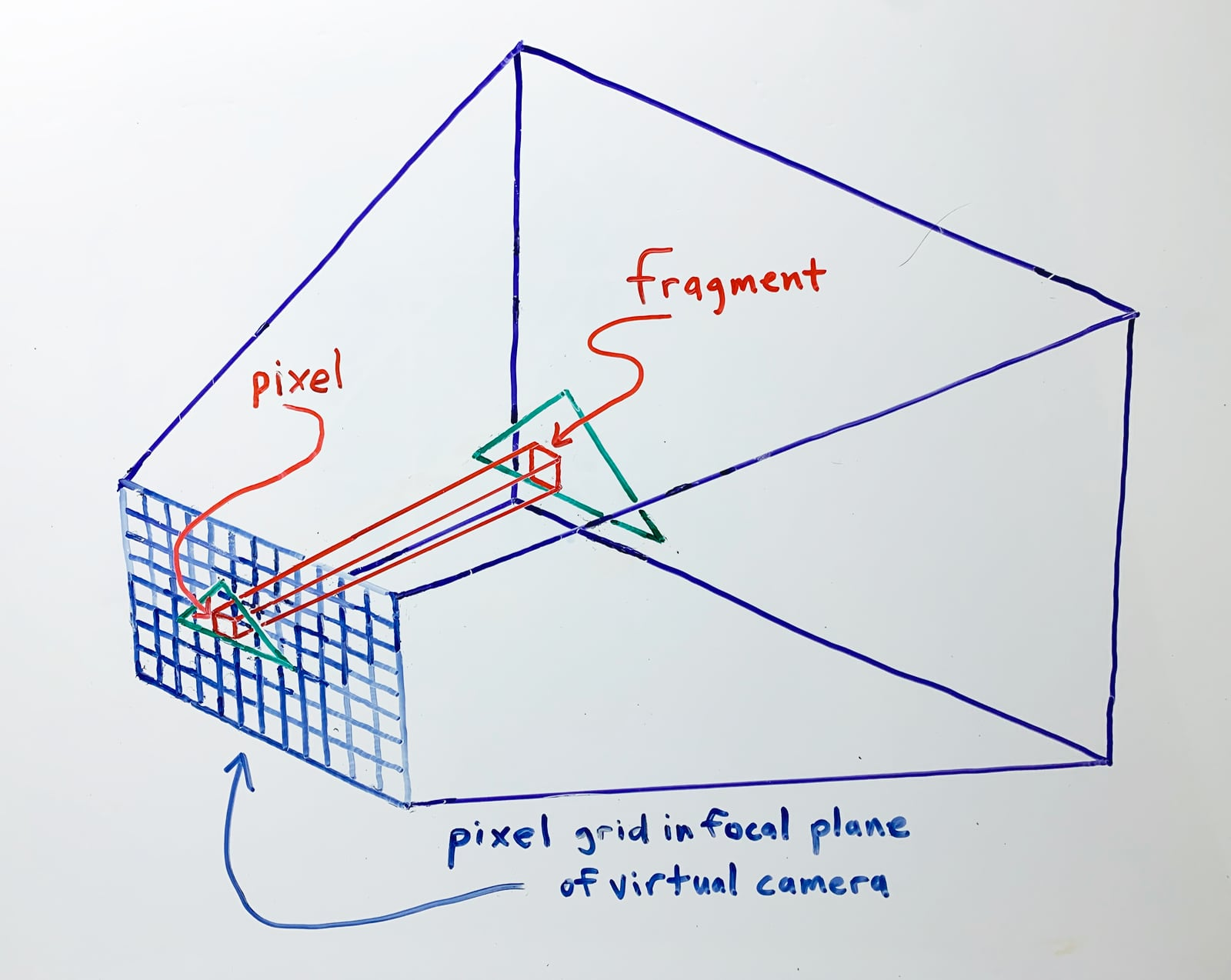Why does the color of each fragment need to be computed? Isn’t the color of a fragment an inherent property of the triangle it belongs to? In a simple sense, yes: a triangle that’s part of the fender of a red car should be colored red. That fact can be recorded as metadata attached to that triangle’s vertices by the creator of the car model.

But in the real world, a red car isn’t always the same shade of red. In bright sunlight, it’s bright red. In shadow, it’s dark red. At night, it might be closer to dark gray. When it’s dirty, it’s a dull, brownish-red. And if the paint is very glossy, it could be reflecting an image of nearby orange construction sign. The more realistic we want our picture of a scene with the red car model to be, the more likely a fragment belonging to a triangle on the car’s fender might need to be colored anything but red! And if the car is driving through a complex environment, that fragment might take on dozens of different colors per second.

The circuitry of a GPU, therefore, is largely dedicated to speeding up the many, many computations required for vertex processing and rasterization. This work is organized into several sequential stages, known collectively as the rendering pipeline. Some of these stages are hard-coded in the GPU’s transistors, but a few can be programmed.

A program for a programmable pipeline stage is called a shader, because historically the first stage that could be programmed was the one that determined fragment colors, a.k.a. shades. The other programmable stages don’t necessarily have anything to do with coloring things, but the name stuck.

The entry point to a fragment shader is a function whose arguments are the attributes of one fragment (including its $$x$$ and $$y$$ coordinates in the bitmap being created), and which returns the color that fragment should be. The wonderful thing about a modern GPU is that this function can be executed for hundreds or thousands of fragments in parallel.

So: what if our 3D scene was simply a rectangle filling the imaging area of the virtual camera? In that case, rasterization is straightforward: the rectangle is decomposed into one fragment for each pixel in the image being created. A fragment shader would therefore be executed exactly once for each pixel. Depending on the GPU, the colors of hundreds or thousands of pixels could be computed simultaneously.

And if, instead of determining the color of a tiny portion of the fender of a red car driving through a city on a rainy day, the shader program happened to compute the boundedness of the iterated Mandelbrot function for the complex point corresponding to the given pixel, rasterization would draw a picture of the Mandelbrot set. Cool! Let’s do this.

How do we write code that runs on a Mac or iOS device’s GPU? OpenGL is a cross-platform library that presents an API for doing that, and it has been available on Apple platforms for many years. But in 2014 Apple introduced Metal, a lower-level API which is optimized for Apple hardware. It should give us the best performance possible on an Apple device, so let’s try it.

Fire up Xcode5 and choose File->New->Playground. Select a blank macOS template.

I like setting the playground to run automatically, which you can do by clicking and holding on the triangular Execute Playground button in the lower left corner of the window. I also like hitting Command-0 to hide the navigator pane. That gives maximum space for code and Mandelbrot set renderings.

Replace the contents of the template with

import MetalKit
import PlaygroundSupport


MetalKit provides the class MTKView, which makes it pretty easy to use Metal to draw into a view that can be arranged with other views in an app. PlaygroundSupport provides an API to render a view in a playground.

First, we’ll ask Metal for a reference to the default GPU device. If we can’t get that for some reason, there’s no point in continuing. If all is well, we’ll print the default GPU’s name to verify it’s what we expect.

guard let device = MTLCreateSystemDefaultDevice() else {
fatalError("Unable to access a GPU.")
}
print(device.name)


On my MacBook Pro, I see

AMD Radeon Pro 555X


Next, we’ll create the MTKView into which we’ll ultimately draw the Mandelbrot set.

let frame = CGRect(x: 0, y: 0, width: 800, height: 800)
let metalView = MTKView(frame: frame, device: device)


Note that metalView references the device object we just created.

800 × 800 pixels is the largest square view I can see all of, side-by-side with the code, on my screen. Feel free to make yours bigger or smaller, but keep it square for now.

Whatever the resolution, we’re going to draw the same view of the Mandelbrot set: all of it, like in our initial console plots. We know from those plots that the set lives between about $$-2.0$$ and $$0.5$$ on the real axis, and $$1.0i$$ and $$-1.0i$$ on the imaginary axis. Our MTKView is square, so let’s make the imaginary axis values range from $$-1.25i$$ to $$1.25i$$, so they also span 2.5 units.

The fragment shader has access to the coordinates of the pixel corresponding to the fragment for which it was invoked. To perform the Mandelbrot set membership test for the complex point corresponding to that pixel, we need to convert from pixel coordinates to complex plane coordinates. The pixel coordinates are in Metal’s viewport coordinate system. This system describes a rectangular 2D space whose origin is at the upper left, with x increasing horizontally to the right, and y increasing vertically downward. If we know what portion of the complex plane is covered by each pixel—the horizontal and vertical stride of a pixel—plus the minimum real value and maximum imaginary value we intend to plot, we can compute the complex point $$c$$ corresponding to each pixel like so (in a c-like pseudocode):

    realPartOfC = minimumReal + (horizontalStride * x)
imaginaryPartOfC = maximumImaginary - (verticalStride * y)


Here’s a diagram showing the same example point in both Metal’s viewport coordinates (where the view is 1000 × 1000 pixels), and in the portion of the complex plane we want to plot.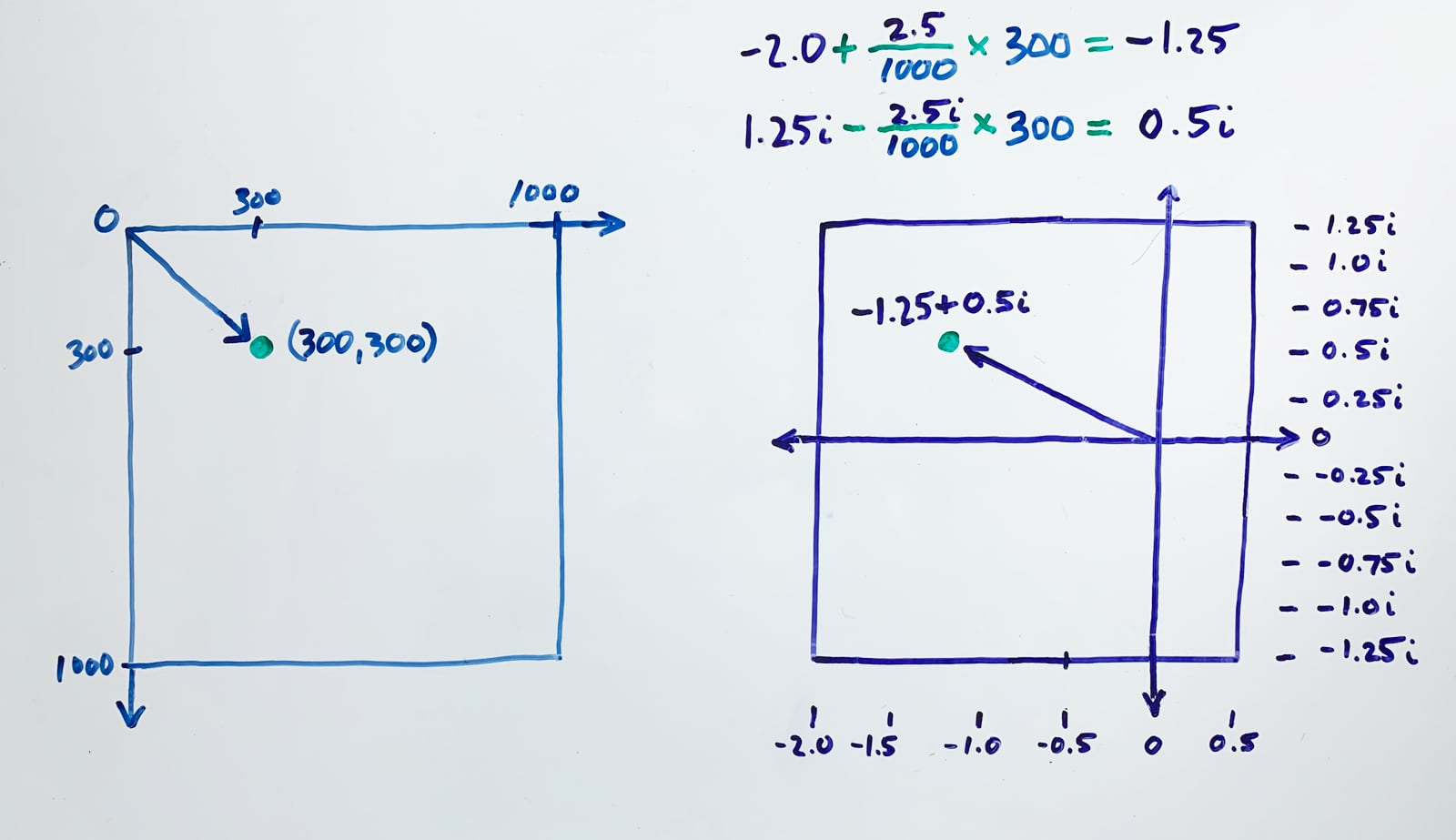Let’s create a new type in Swift to hold the information we need to make this coordinate space conversion.

struct ViewParams {
let minimumReal: Float
let maximumImaginary: Float
let horizontalStride: Float
let verticalStride: Float
}


Use the new type to make a value reflecting the view we want to draw.

let lowerLeft = simd_float2(x: -2.0, y: -1.25)
let upperRight = simd_float2(x: 0.5, y: 1.25)
var viewParams = ViewParams(
minimumReal: lowerLeft.x,
maximumImaginary: upperRight.y,
horizontalStride: (upperRight.x - lowerLeft.x) / Float(frame.width),
verticalStride: (upperRight.y - lowerLeft.y) / Float(frame.height)
)
print(viewParams)


Okay, what’s all this now? What’s a simd_float2?

The SIMD vector types allow for Single Instruction, Multiple Data computation on Apple platforms. SIMD improves performance by allowing computation on several pieces of data within one machine language instruction. SIMD vector types are used extensively in Metal, and we’ll see more of them when we start writing shader code.

But they’re not being used above for their performance benefits. We simply want a type that describes a 2D point with a Float for each coordinate, and which can be used in both Swift and shader code. simd_float2 provides exactly that.

For an 800 × 800 view, the printed params are

ViewParams(minimumReal: -2.0, maximumImaginary: 1.25, horizontalStride: 0.003125, verticalStride: 0.003125)


That looks good: the horizontal and vertical stride are equal, as we’d expect, since we’re drawing a square portion of the complex plane into a square view.

We computed these params in Swift, where we have access to the view bounds. But we need to use them in the fragment shader. Metal has APIs which we’ll meet shortly that make Swift objects accessible to code running on the GPU.

There’s another object we need to construct in Swift and use in shader code: our only model, the rectangle filling the virtual camera’s view. Metal can’t draw a rectangle directly, so we’ll have to build one out of two right triangles. We define these triangles by specifying the coordinates of their six vertices.

To do that, we need to know what coordinate system the triangles will live in. That turns out to be Metal’s normalized device coordinate system. This is a 3D space which is 2 units wide, ranging from $$-1.0$$ to $$1.0$$ along the $$x$$ axis; 2 units high, from $$-1.0$$ to $$1.0$$ along the $$y$$ axis; and 1 unit deep, from $$0$$ to $$1.0$$ along the $$z$$ axis—regardless of the size of the view that Metal is rasterizing into. The focal plane of the virtual camera is defined by $$z = 0$$, so points with increasing $$z$$ values are increasingly far from the camera.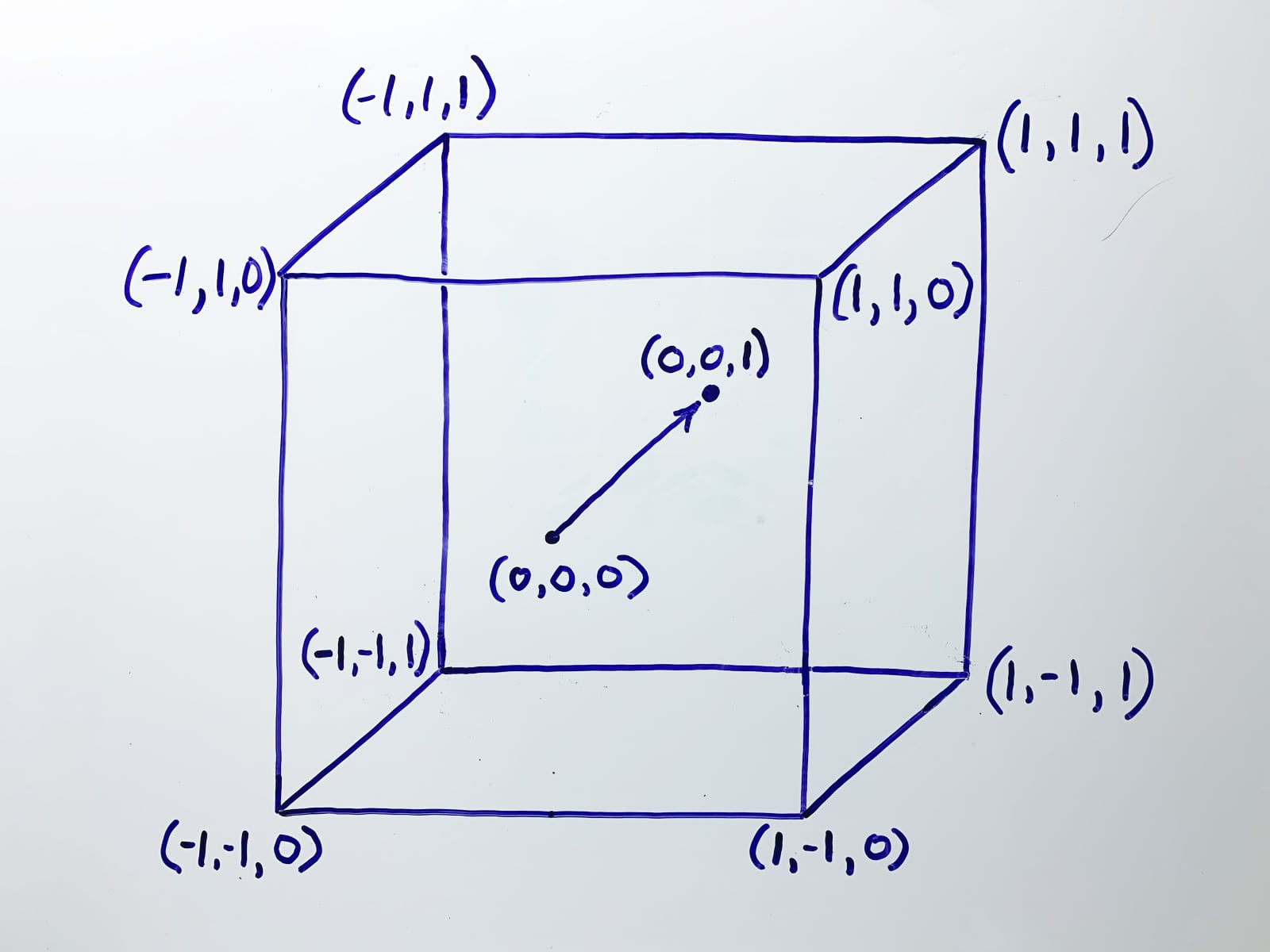That means the upper left corner of our desired rectangle is at $$(-1.0, 1.0, 0)$$, and its lower right corner is at $$(1.0, -1.0, 0)$$. We compose it from two triangles like so: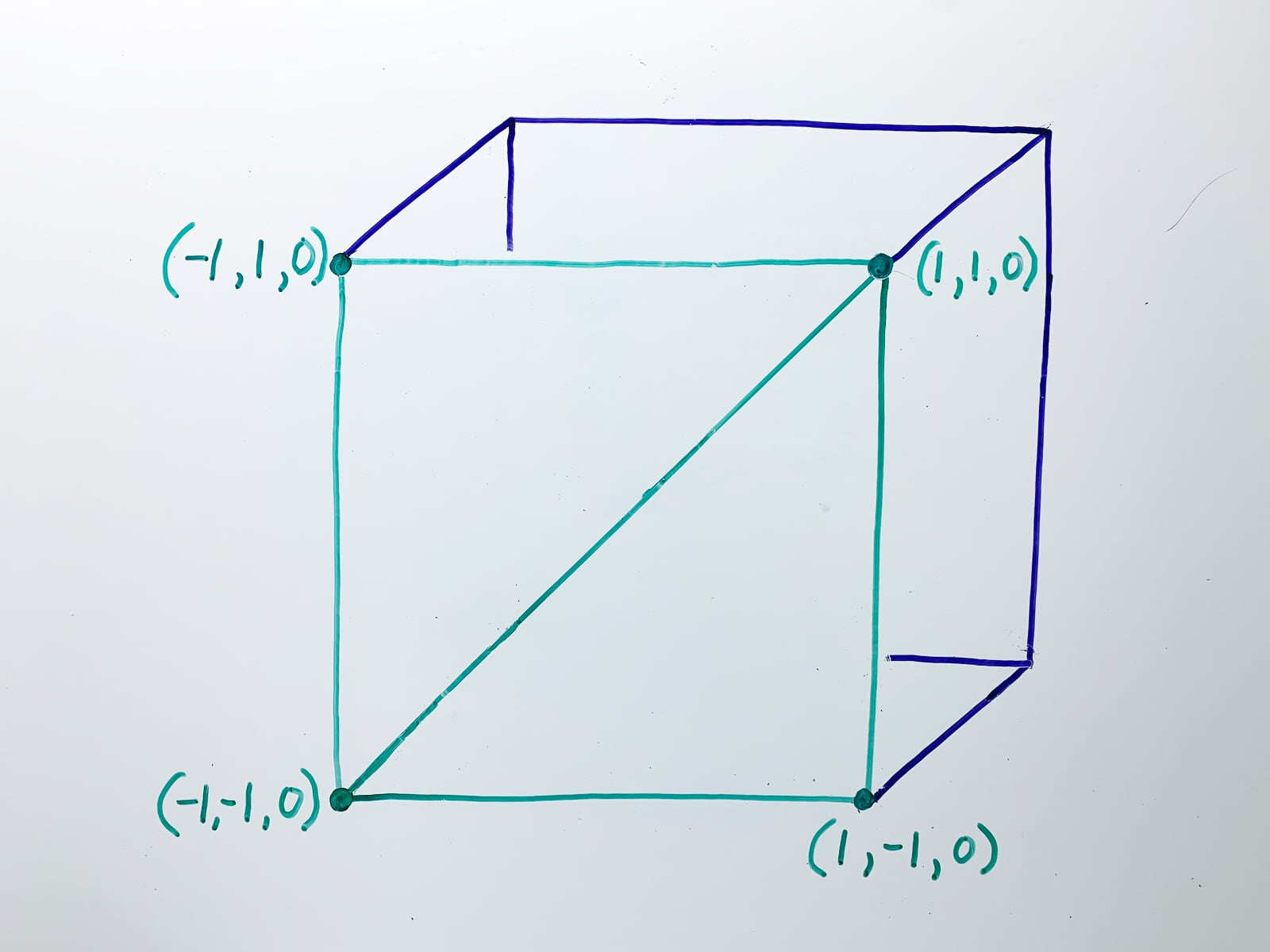In Swift, that’s

let positions: [simd_float4] = [
simd_float4(-1.0, 1.0, 0.0, 1.0),
simd_float4(1.0, 1.0, 0.0, 1.0),
simd_float4(-1.0, -1.0, 0.0, 1.0),
simd_float4(1.0, 1.0, 0.0, 1.0),
simd_float4(1.0, -1.0, 0.0, 1.0),
simd_float4(-1.0, -1.0, 0.0, 1.0)
]


Vertex positions must be converted to Metal’s normalized device coordinates, and be of type simd_float4, before they can be handed off to the rasterizer. If we were loading vertices from a model designed in a 3D modeling tool, their coordinates would likely be expressed in a different coordinate space, and we would eventually have to convert them—preferably in the GPU, to take advantage of its high computational performance. But we’re defining only a few coordinates, right here in Swift, so we can express them directly in the coordinate space and format Metal requires.

Why simd_float4? Metal needs to perform lots of computations on vertex positions, much of which take the form of matrix multiplications, which can be accelerated by SIMD.

But why four floats for a 3D coordinate? It’s due to the mathematics of matrix multiplication, which requires that the number of columns in the first matrix matches the number of rows in the second matrix. A 3D point can be exactly represented by a 3-element vector, which is also a 3 × 1 or 1 × 3 matrix (take your pick). A point can be rotated about the origin, scaled, or translated (moved) by multiplying the vector of its three coordinates with a 3 × 3 matrix. But if a fourth coordinate with a value of $$1.0$$, conventionally named $$w$$, is added to the position vector, then it can be multiplied against a 4 × 4 matrix. That allows the point to be both rotated or scaled and translated with only one multiplication. A coordinate space with an extra dimension to enable more powerful computation is a homogenous coordinate system.

We now have two pieces of data we’d like to make accessible to shader code. Each needs a unique index we can use to refer to them. Let’s use 0 as the index of the vertex list, and 1 as the index of the view params we defined earlier.

It’s time to write some shaders! As we discussed, all the work of computing the boundedness of the iterated Mandelbrot function for each complex point will be done in a fragment shader. Metal requires us to also write a vertex shader, which we haven’t yet talked about.

Just as a fragment shader is executed once for each fragment, a vertex shader is executed once for each vertex. It’s typically used to transform vertex coordinates from model space, whose origin is relative to a particular model, to world space, whose origin is relative to the entire scene, to view space, whose origin is relative to the virtual camera. A vertex shader also has access to any other vertex attributes that may exist—for example, the inherent color of the vertex’s parent triangle—so it might also perform calculations that aren’t strictly about geometry. But as our 3D scene comprises just one rectangle that fills the entire imaging area of the virtual camera, and we’ve already defined its vertex positions as required by Metal, we have little use for a vertex shader. Ours will simply pass through each position unmodified.

Metal shaders are written in Metal Shading Language (MSL), which is based on C++. Don’t worry if you don’t know C++—the shaders we’ll write won’t use any fancy language features, and I’ll explain everything we type.

In a typical Metal application project, you’d write your shaders in separate files named with the .metal extension. Since we’re working in a playground, we’ll define our shaders right in our Swift code, using a multiline string literal.

let shaders = """


In case you haven’t seen it before, """ starts a Swift multiline string literal. The next """ indented to the same level will end it.

Now we can write some Metal! MSL, that is. First, a bit of boilerplate which looks suspiciously like C++.

#include <metal_stdlib>
using namespace metal;


We include the header for Metal’s standard library, and declare that we would like to use entities defined in the metal namespace without having to prefix each one with metal::.

Next, we define the return type of our vertex function.

struct VertexOut {
float4 position [[position]];
};


As in Swift, struct defines a new type, named, in this case, VertexOut. This type has only one data member of type float4, named position. float4 is an MSL type compatible with Swift’s simd_float4.

[[position]] is a thing you may not have seen before if (like me!) you haven’t written C++ for a while: it’s an example of an attribute. Attributes were introduced in C++11 as a standard way for different C++ implementations to define their own extensions to the language. And MSL definitely needs to define some of its own extensions, because it runs in the unique environment of a GPU, rather than on a general purpose CPU.

As I mentioned before, a sophisticated renderer might attach all sorts of additional metadata to each vertex—for example, a color. That would be accomplished by adding additional members to VertexOut. But whatever else VertexOut might contain, it must have the coordinates of the vertex, because they need to be passed to the next stage of the rendering pipeline. The [[position]] attribute tells the compiler which struct member holds the coordinates of the vertex.

Now we can define the vertex shader function.

[[vertex]] VertexOut
vertex_main(
device const float4 * const positionList [[buffer(0)]],
const uint vertexId [[vertex_id]]
) {
VertexOut out {
.position = positionList[vertexId],
};
return out;
}


The [[vertex]] attribute tells the compiler that the following function is a vertex shader entry point. The function’s return type is the VertexOut struct we just defined, and the function’s name is vertex_main.

The function’s parameter list follows, inside parentheses. Let’s tackle the many parts of the first parameter declaration. positionList is the name we’ve given to the parameter. Its type is float4 *, which means it’s a pointer to the location in memory of a float4. This particular float4 is the first vertex position in the list we defined in Swift as an array of simd_float4. In C++ and MSL, arrays that are allocated in a memory heap (rather than on the stack) are accessed through pointers. So MSL’s type float4 * is used to access the storage of a Swift [simd_float4].

In C++, I like to apply const to the declaration of every variable and parameter whose value I don’t expect to change during its lifetime. That includes writing const after * when I don’t expect the address of a pointer to change. I like the compiler to complain if my assumption of immutability is wrong. I’ve carried that habit over to MSL.

Metal defines several distinct address spaces in which memory can be allocated. device tells the compiler that memory for the argument to the following parameter is allocated from the space in which we can make Swift objects accessible. We haven’t yet written the code to do that. We’ll get around to it after we’ve finished the shaders. But we have decided to use the index 0 to refer to the list of vertices.

And that’s what [[buffer(0)]] is for: it tells the compiler that it should pass a pointer to the zeroth item in the buffer of things made accessible from Swift as the argument for this parameter.

The second parameter, named vertexID, identifies the vertex for which the function has been called. We’re sending the GPU six vertices, so we should expect this vertex function to be called six times (in parallel!), with the values 0 through 5 passed for this parameter. The attribute [[vertex_id]] tells the compiler to do just that.

The body of our vertex function is simple: create a VertexOut value, set its position member to the position in the list of positions identified by vertexId, and return it.

We’ve actually done a bit more work than we needed to. The only requirement of the vertex shader function is that it return the position in the normalized device coordinates system as a float4. In our simple case, where we don’t need to attach any additional attributes to our vertices, we could have just returned the position directly, instead of wrapping it in the VertexOut type.

Next up: the fragment shader, where all the magic will happen! First, we need to declare our ViewParams type in MSL.

struct ViewParams {
float minimumReal;
float maximumImaginary;
float horizontalStride;
float verticalStride;
};


If we were working in a project, instead of a playground, we could have defined this type once in a C++ header file shared between Swift and MSL. The Swift compiler is able to generate Swift types from compatible C++.

Now, the fragment shader function itself.

[[fragment]] float4
fragment_main(
const VertexOut in [[stage_in]],
constant ViewParams &viewParams [[buffer(1)]]
) {


Similar to [[vertex]], the [[fragment]] attribute tells the compiler that the following is a fragment shader function. In this case, it’s a function named fragment_main which returns a float4—which will be the computed red-green-blue-alpha color of the fragment.

fragment_main takes two parameters. The first, named in, is of the type VertexOut that we returned from our vertex function. But there’s a crucial difference in the data: the coordinates are no longer in normalized device space, but rather in viewport space. That means x and y are the coordinates of the pixel whose color we want to calculate. The [[stage_in]] tells the compiler to obtain the value of this parameter from the data passed by the GPU to this stage of the pipeline.

The second fragment function parameter, viewParams, contains a reference (indicated by &) to the location in memory of the view parameters we defined in Swift. In C++ and MSL, a reference is like a constant pointer, in that it can only ever refer to one location in memory, but it’s more restricted in that you can’t perform arithmetic on it to access adjacent objects of the same type. We’ll eventually place the view parameters into the second zero-based location in the buffer of things made accessible from Swift, so we give viewParams the [[buffer(1)]] attribute.

What is constant? It’s not a typo of const. Like device, which we applied to the positionList parameter of the vertex function, constant tells the compiler that the object referenced by viewParams lives in the buffer of things made accessible from Swift. But this object is accessed differently than the list of vertex positions. viewParams is a single object bundling four floats, which is read concurrently by many shader function instances executing in parallel. The constant buffer is optimized for this usage. To ensure constant buffer data is consistent for each shader function instance, the compiler enforces read-only access to objects that live in it. In contrast, positionList contains six different bundles of four floats, and each bundle is only ever read by one shader function instance. The device buffer is optimized for that access pattern.

We begin the body of the fragment function by converting the pixel coordinates passed in in.position to complex plane coordinates, using the equations we derived above.

    const float2 c = float2(
viewParams.minimumReal + in.position.x * viewParams.horizontalStride,
viewParams.maximumImaginary - in.position.y * viewParams.verticalStride
);


Now we iterate the Mandelbrot function to determine its boundedness for $$c$$. In other words, whether

$\lvert z_n \rvert <= 2$

for some number of iterations of

$z_{n+1} = z_n^2+c$

where $$z_0 = 0$$.

    float2 z = float2(0, 0);
const uint maxIterations = 30;
uint i = 0;

while (i < maxIterations) {
float2 zSquared = float2(z.x*z.x - z.y*z.y, z.x*z.y + z.y*z.x);
z.x = zSquared.x + c.x;
z.y = zSquared.y + c.y;
++i;
if ((z.x*z.x + z.y*z.y) > 4.0f) {
break;
}
}


This is a pretty straightforward implementation, with one optimization: instead of comparing the length of z with 2.0, which requires making an expensive square root computation, we compare the squared length with 4.0. We’ll investigate optimization more fully in a future blog post.

Now that we’ve determined boundedness, all that’s left is to color this pixel! Similar to when we plotted the set in the console, let’s choose black for set members, and white for values of c that make z blow up. The color is specified by returning a float4 whose members indicate the red, green, blue, and alpha component values, respectively.

    if (i >= maxIterations) {
return float4(0, 0, 0, 1);
} else {
return float4(1, 1, 1, 1);
}
}


Finally, we need to terminate this multiline Swift string.

"""


If that’s your first Metal Shading Language code, congratulations! All that’s left before seeing it in action is configuring and running Metal’s rendering pipeline.

Create a MTLRenderPipelineDescriptor. This is a container for various bits of pipeline configuration data.

let pipelineStateDescriptor = MTLRenderPipelineDescriptor()


Use it to set the in-memory format of the pixels of the images created during rasterization. .bgra8Unorm means a 32-bit value comprising 8 bits each for blue, green, red, and alpha (transparency), in that order.

pipelineStateDescriptor.colorAttachments.pixelFormat = .bgra8Unorm


The descriptor also tells Metal where to find our shader code, so it can compile it into the pipeline state. Recall that our shader source is in the multiline string we assigned to the shaders constant.

let library = try! device.makeLibrary(source: shaders, options: nil)
let vertexFunction = library.makeFunction(name: "vertex_main")
let fragmentFunction = library.makeFunction(name: "fragment_main")
pipelineStateDescriptor.vertexFunction = vertexFunction
pipelineStateDescriptor.fragmentFunction = fragmentFunction


That’s all the pipeline configuration we need. Now we ask Metal to construct a pipeline state object from the descriptor. This will compile the shaders and perform other potentially expensive operations. Note that the descriptor only allows setting one vertex and one shader function. A more complex renderer which requires several different shaders would create a separate pipeline state for each pair of shaders at startup, and then switch between these pre-constructed states as needed.

let pipelineState = try! device.makeRenderPipelineState(descriptor: pipelineStateDescriptor)


To make the GPU draw stuff in our MTKView, we send it drawing commands. A GPU is a shared resource—there are many concurrently-running apps that want it to draw stuff for them. Most of the macOS and iOS UI is rendered by the GPU, so it will still be receiving commands to draw bits of Xcode’s windows while we ask it to draw the Mandelbrot set. To manage these competing requests, Metal requires apps to put their drawing commands into queues, which it then processes in an orderly fashion.

Specifically, commands are encoded into a command buffer, which belongs to a command queue. Let’s create these objects.

guard let commandQueue = device.makeCommandQueue(),
let commandBuffer = commandQueue.makeCommandBuffer(),
let descriptor = metalView.currentRenderPassDescriptor,
let commandEncoder = commandBuffer.makeRenderCommandEncoder(descriptor: descriptor),
let drawable = metalView.currentDrawable else {
fatalError("Problem setting up to draw a frame.")
}


We created a command queue for our device, a command buffer for that queue, and a command encoder for that buffer. We also made sure that metalView actually has a memory buffer to draw into, which we can now refer to as drawable.

There’s one last step before we can use the command encoder: we tell it what pipeline state we want to be in effect for the commands we’re going to send it.

commandEncoder.setRenderPipelineState(pipelineState)


Now that the command encoder is initialized, we can use it to make the list of vertex positions available to the vertex shader, and the view params object accessible to the fragment shader. Metal provides multiple ways to do that, each with various tradeoffs between ease of use, performance, and efficiency. This is a topic I need to learn more about, so I won’t make claims we’re about to do this “the right way”. And we only need to ask the GPU to draw one frame in order to see the Mandelbrot set, so high performance is not currently a concern (though it will be in a future post, when we start panning around and zooming into the set!). For now, we’ll just demonstrate two of the possible ways.

For objects that are greater than 4 KB in size, or which are expected to persist over the lifetime of many commands, we can construct a MTLBuffer to contain the object, using MTLDevice’s makeBuffer method, and then pass the buffer to MTLCommandEncoder’s setVertexBuffer or setFragmentBuffer method to make it accessible to the vertex or fragment shader, respectively. There’s some overhead in creating the buffer, which is why this method is better for cases where we can construct the buffer once and use it for a while, instead of cases where the buffer would have to be constructed repeatedly in a tight loop. That makes this option good for model data, like our list of vertex positions. We only have six vertices, but a serious 3D renderer like a game engine has millions or billions. And model data typically persists for many frames of animation, and thus many drawing calls.

To determine the correct size for the buffer, we’ll use the handy MemoryLayout generic enumeration. When instantiated for a type, it provides information about the size of instances of that type. In particular, we’ll use the stride member to get the size an instance occupies in memory, including any padding.

let positionLength = MemoryLayout<simd_float4>.stride * positions.count
let positionBuffer = device.makeBuffer(bytes: positions, length: positionLength, options: [])!
commandEncoder.setVertexBuffer(positionBuffer, offset: 0, index: 0)


Note that we set the index of the position list to 0, as we decided previously.

For smaller objects, or ones that only live as long as one command or frame, we can use MTLCommandEncoder’s setVertexBytes or setFragmentBytes, which eliminate the overhead of creating a MTLBuffer object. Let’s try this with our view params object.

commandEncoder.setFragmentBytes(&viewParams, length: MemoryLayout<ViewParams>.stride, index: 1)


Now all that’s left is to tell the GPU to draw some triangles, using the list of vertex positions, into metalView’s drawable.

commandEncoder.drawPrimitives(type: .triangle, vertexStart: 0, vertexCount: positions.count)

commandEncoder.endEncoding()

commandBuffer.present(drawable)
commandBuffer.commit()


Committing the command buffer schedules the commands it contains for execution. To see the results, we set our Metal view as the live view for the current playground page.

PlaygroundPage.current.liveView = metalView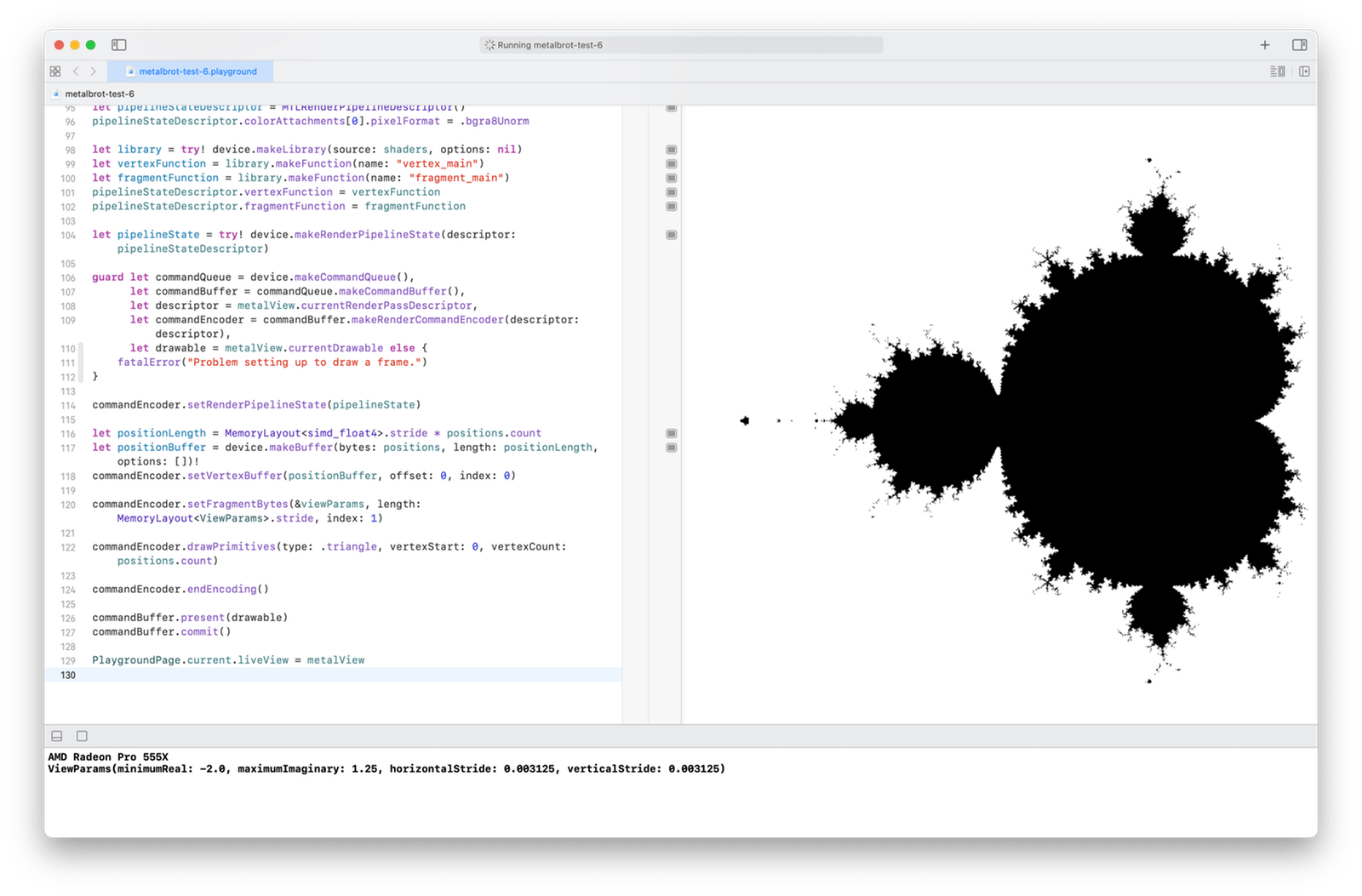And there’s a sharper image of the Mandelbrot set! It’s now sampled at hundreds of thousands of points, instead of around ten thousand like we did in the console. We see quite a bit more of the fascinating complexity of the set border that was only hinted at in our console plots.

But if you’ve seen pretty pictures of the set before, you may lament our rendering’s absence of color. We’re only testing whether a point is in or out of the set, so we only have two states to map to colors. We’ve chosen black and white, but choosing orange and blue or chartreuse and periwinkle won’t get us to those dazzling images we’ve seen on the internet. How do they—and how can we—render the set with a palette of more than two colors?

Well, there’s only one way a point can be in the set: when it’s plugged into the Mandelbrot function as $$c$$, repeatedly iterating the equation should not cause the sequence of function values to blow up.

But there are lots of ways a point can be out of the set. It can make the function values blow up very quickly, after just a few iterations. Or it can require many, many iterations. We learned when we studied the emergence of $$\pi$$ in the set that as a point gets closer and closer to the set’s amazing fractal border from the outside, it can take billions of iterations for the length of $$z$$ to finally exceed $$2.0$$. A point infinitesimally close to the border means $$z$$’s magnitude is bounded after an infinity of iterations—which is the same thing as being in the set. Don’t quote me; I’m not a mathematician. Anyway, the count of iterations to blowup is a measure of a point’s closeness to the set, and we can visualize that closeness by assigning a different color to each count.

We’ll leave full color for a future blog post, but with a simple tweak to our fragment shader, we can at least add shades of gray. Change the last part, where we return the color white for non-set-members, like so:

    if (i >= maxIterations) {
return float4(0, 0, 0, 1);
} else {
//        return float4(1, 1, 1, 1);
float normalized = float(i) / (maxIterations - 1);
return float4(normalized, normalized, normalized, 1);
}


Instead of returning white, we now scale the iteration count to a real value between float(1) / (maxIterations - 1) and 1.0. Setting the red, green, and blue components of the color to that same value maps the iteration count to a range of grays: from just a bit lighter than black, to white, since the iteration count in the blowup case will range from 1 (we increment the counter at least once before testing) to maxIterations - 1. The brightest shades are closest to the set border, with full white right next to the pure black of the set members for some nice contrast.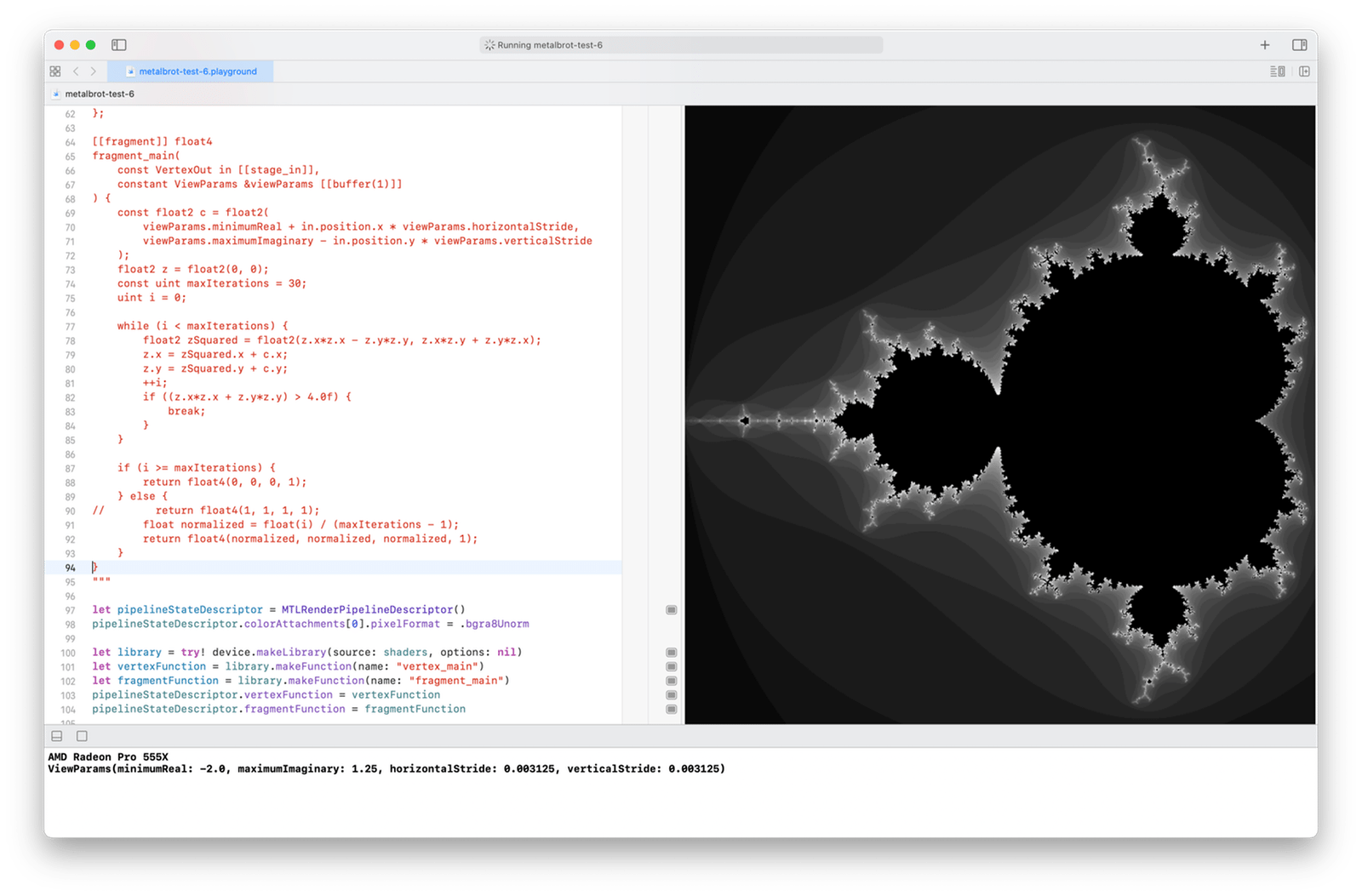That’s more like it!

Try changing maxIterations in the fragment shader to find the rendering you like best. My current favorite value is 60, which shows more detail in the border.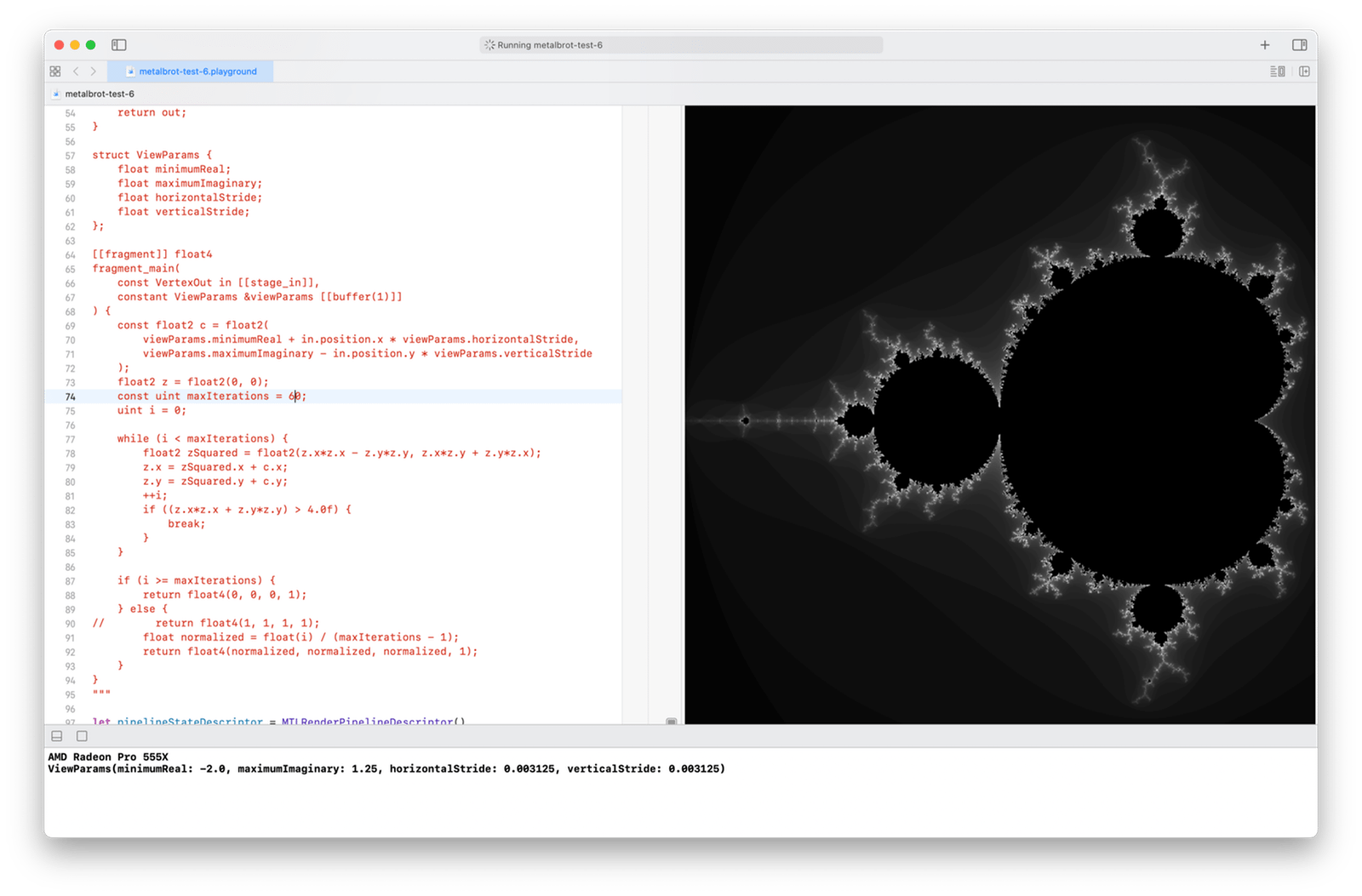Choosing an ideal color mapping is certainly subjective, but there’s also some subtle science to it. Our view is zoomed out enough to show the whole set, including a lot of points quite far from the set that cause blowup on the first iteration. For example, $$c = -1.75 - 1.0i$$ is close to the lower left corner of our picture, and has a magnitude of just over $$2.0$$.

As we increase maxIterations, the picture gets darker and darker. At 1000, the set border is barely visible.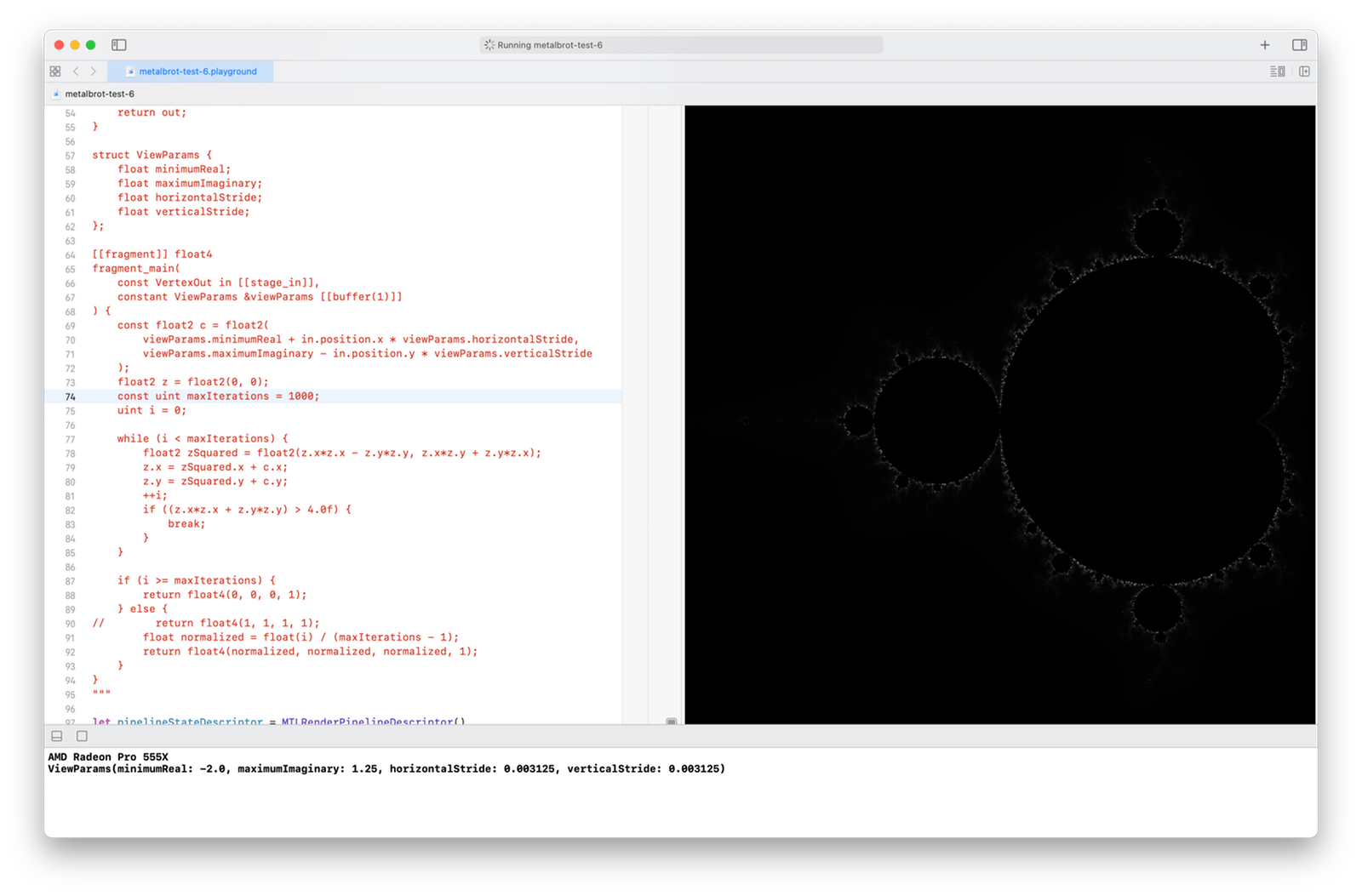This is because there are very few points in our zoomed out view, if any, that are close enough to the set border to require 1000 iterations before blowup. We have a thousand colors in our palette, but we’re using only a fraction of them, all on the darker side. Our color mapping needs to be adjusted to zoom level, as well as to our tastes.

I hope to explore color mapping further in a future post. But first, I want to tackle panning and zooming—in a real app! Stay tuned.

#### Revision History

• 2020-12-15: Improved and expanded the discussion of shader function parameters. It’s now more rigorous (for example, I describe the constant address space and compare it to device, instead of ignoring it), and less wrong (I now explain the semantics of const float4 * correctly—I think).

1. Or “delightfully parallel”. I can’t decide which I love more.

2. GPU cores are more specialized than a traditional CPU’s general-purpose cores. Happily, what they specialize in is exactly what we need: high-performance numerical computation.

3. I learned most of what I currently know about 3D graphics hardware and software from raywenderlich.com’s 3D Graphics with Metal video course and their book Metal by Tutorials, Second Edition, and Jason Gregory’s Game Engine Architecture, Second Edition. Happily, I still have much yet to learn!

4. I’m using Xcode 12.3 on macOS Big Sur 11.1.